# How to use the PCAmixdata Package

## PCAmix

The datatable housing is used.

``````library(PCAmixdata)
data(gironde)
``````##                    density primaryres   houses owners council
## ABZAC               131.70      88.77  inf 90%  64.23  sup 5%
## AILLAS               21.21      87.52  sup 90%  77.12  inf 5%
## AMBARES-ET-LAGRAVE  531.99      94.90  inf 90%  65.74  sup 5%
## AMBES               101.21      93.79  sup 90%  66.54  sup 5%
## ANDERNOS-LES-BAINS  551.87      62.14  inf 90%  71.54  inf 5%
## ANGLADE              63.82      81.02  sup 90%  80.54  inf 5%``````

The PCAmix function is applied to this datatable.

``````split <- splitmix(gironde\$housing)
X1 <- split\$X.quanti
X2 <- split\$X.quali
res.pcamix <- PCAmix(X.quanti=X1, X.quali=X2,rename.level=TRUE,
graph=FALSE)``````

The object res.pcamix is an object of class PCAmix and contains many numerical results summarized in the S3 print method.

``res.pcamix``
``````##
## Call:
## PCAmix(X.quanti = X1, X.quali = X2, rename.level = TRUE, graph = FALSE)
##
## Method = Principal Component of mixed data (PCAmix)
##
##
## "name" "description"
## "\$eig" "eigenvalues of the principal components (PC) "
## "\$ind" "results for the individuals (coord,contrib,cos2)"
## "\$quanti" "results for the quantitative variables (coord,contrib,cos2)"
## "\$levels" "results for the levels of the qualitative variables (coord,contrib,cos2)"
## "\$quali" "results for the qualitative variables (contrib,relative contrib)"
## "\$coef" "coef of the linear combinations defining the PC"``````

The variance of the principal components is obtained.

``res.pcamix\$eig``
``````##       Eigenvalue Proportion Cumulative
## dim 1  2.5268771  50.537541   50.53754
## dim 2  1.0692777  21.385553   71.92309
## dim 3  0.6303253  12.606505   84.52960
## dim 4  0.4230216   8.460432   92.99003
## dim 5  0.3504984   7.009968  100.00000``````

### Graphical outputs

Here are some graphical outputs obtained with the method plot of the objects of class PCAmix.

``?plot.PCAmix``
``## Rendering development documentation for 'plot.PCAmix'``
``````par(mfrow=c(2,2))
plot(res.pcamix,choice="ind",coloring.ind=X2\$houses,label=FALSE,
posleg="bottomright", main="Observations")
plot(res.pcamix,choice="levels",xlim=c(-1.5,2.5), main="Levels")
plot(res.pcamix,choice="cor",main="Numerical variables")
posleg="topright", main="All variables")``````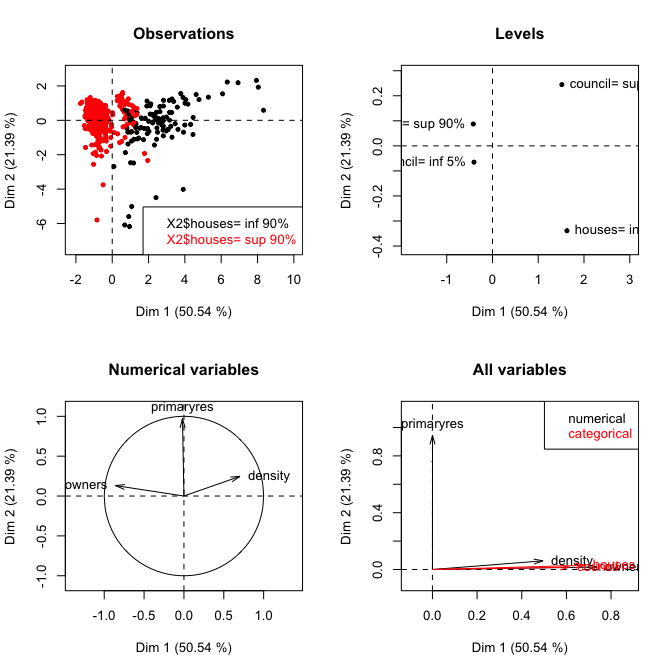It is also possible to select some observations to plot.

``sort(res.pcamix\$ind\$coord[,2])[1:10]``
``````##  VENDAYS-MONTALIVET             CARCANS             LACANAU
##           -6.171971           -6.087304           -6.070451
##      SOULAC-SUR-MER GRAYAN-ET-L'HOPITAL     LEGE-CAP-FERRET
##           -5.802359           -5.791642           -5.596315
##      VERDON-SUR-MER             HOURTIN            ARCACHON
##           -5.008545           -4.493259           -4.013374
##               PORGE
##           -3.751233``````
``````plot(res.pcamix,choice="ind",lim.contrib.plot = 2,
posleg="bottomright", main="Observations",cex=0.8)``````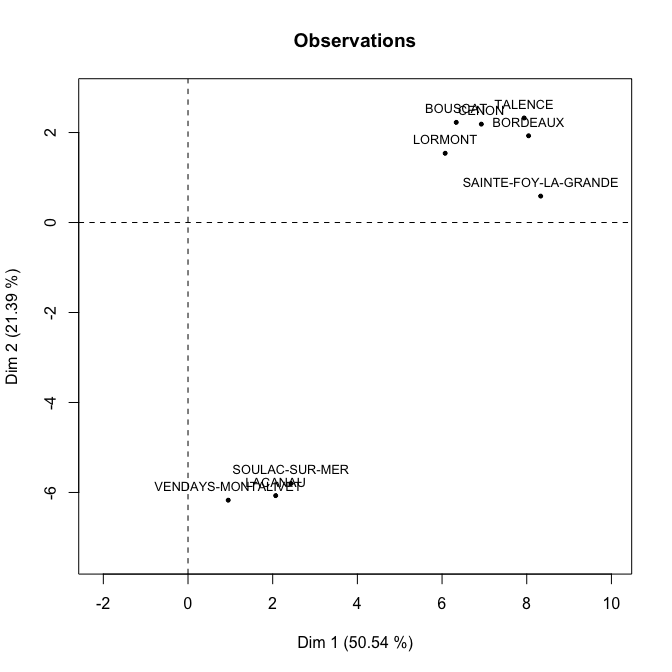### Prediction and plot of new observations.

We select randomly 100 observations to build a test sample. The remaining observations are in a train sample used to perform PCAmix.

``````set.seed(10)
test <- sample(1:nrow(gironde\$housing),100)
train.pcamix <- PCAmix(X1[-test,],X2[-test,],ndim=2,graph=FALSE)``````

The scores of the 100 observations are obtained with the S3 method predict of the objects of class PCAmix.

``?predict.PCAmix``
``## Rendering development documentation for 'predict.PCAmix'``
``````pred <- predict(train.pcamix,X1[test,],X2[test,])
``````##                                dim1        dim2
## MAZION                   -0.4120140  0.03905247
## FLAUJAGUES               -0.6881160 -0.33163728
## LATRESNE                  0.7447583  0.65305517
## SAINT-CHRISTOLY-DE-BLAYE -0.7006372 -0.33216807
## BERSON                   -1.1426625  0.33607088

It is then possible to plot these observation as supplementary on the principal component map.

``````plot(train.pcamix,axes=c(1,2),label=FALSE,main="Observations map")
points(pred,col=2,pch=16)
legend("bottomright",legend = c("train","test"),fill=1:2,col=1:2)``````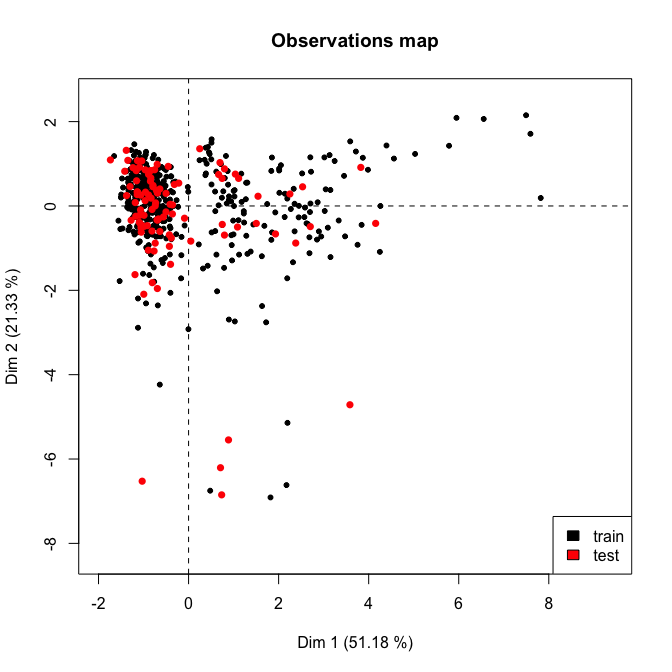### Plot of supplementary variables

We choose as supplementary variables: * the numerical variable building of the datatable environment * the categorical variable doctor of the datatable services And we use the S3 method supvar of the objects of class PCAmix to obtain the coordinates of this supplmentary numerical on the correlation circle of PCAmix as well as the coordinates of the levels of this supplementary categorical variable.

``?supvar.PCAmix``
``## Rendering development documentation for 'supvar.PCAmix'``
``````X1sup <- gironde\$environment[,1,drop=FALSE]
X2sup <- gironde\$services[,7,drop=FALSE]
res.sup <- supvar(res.pcamix,X1sup,X2sup,rename.level=TRUE)
res.sup\$quanti.sup\$coord[,1:2,drop=FALSE]``````
``````##               dim1      dim2
## building 0.6945295 0.1884711``````
``res.sup\$levels.sup\$coord[,1:2]``
``````##                      dim1         dim2
## doctor=0      -0.44403187 -0.006224754
## doctor=1 to 2  0.07592759 -0.112352412
## doctor=3 or +  1.11104073  0.099723319``````

The S3 method plot plots automatically these supplementary variables on all the graphical outputs.

``?plot.PCAmix``
``## Rendering development documentation for 'plot.PCAmix'``
``````#par(mfrow=c(2,1))
plot(res.sup,choice="cor",main="Numerical variables")``````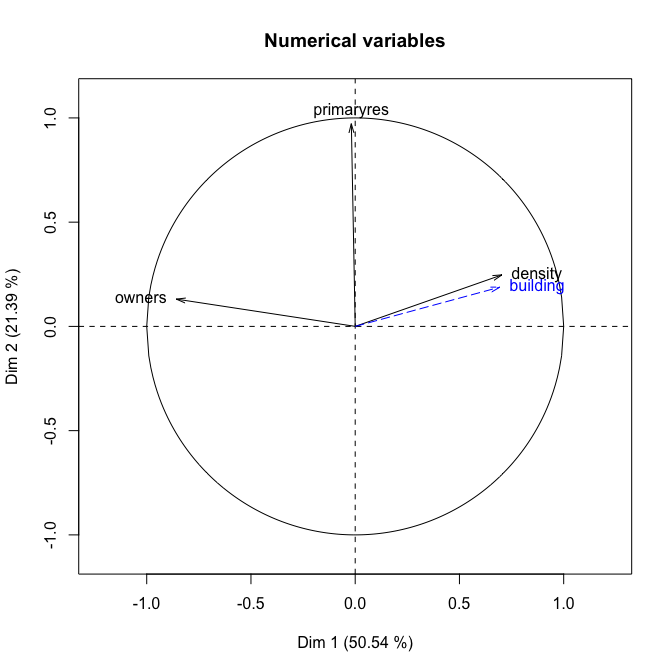``plot(res.sup,choice="levels",main="Levels",xlim=c(-2,2.5))``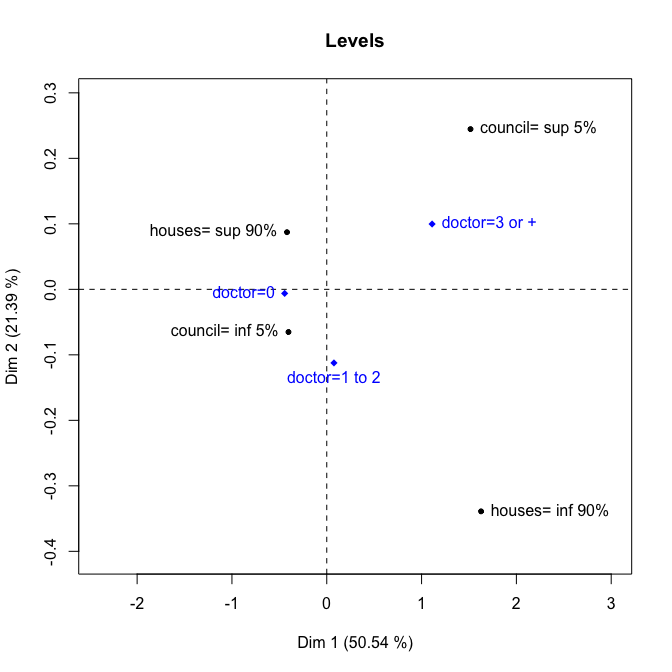## PCArot

We apply here the function PCArot to perform varimax-type rotation to the result of PCAmix applied to the datatable housing. The 10 first observations are removed to illustrate later the prediction of new observations.

``````library(PCAmixdata)
data(gironde)
#Create the datatable housing without the ten first observations
train <- gironde\$housing[-c(1:10), ]
#Split the datatable
split <- splitmix(train)
X1 <- split\$X.quanti
X2 <- split\$X.quali
res.pcamix <- PCAmix(X.quanti=X1, X.quali=X2,rename.level=TRUE,
graph=FALSE)``````

In order to choose the number of dimension to rotate, we look at the inertia of the principal components again.

``res.pcamix\$eig ``
``````##       Eigenvalue Proportion Cumulative
## dim 1  2.5189342  50.378685   50.37868
## dim 2  1.0781913  21.563825   71.94251
## dim 3  0.6290897  12.581794   84.52430
## dim 4  0.4269180   8.538361   93.06267
## dim 5  0.3468667   6.937335  100.00000``````

We decide to rotate 3 principal components that summarize 84% of the inertia.

``````res.pcarot <- PCArot(res.pcamix,dim=3,graph=FALSE)
res.pcarot\$eig #variance of the rotated PCs``````
``````##          Variance Proportion
## dim1.rot 1.919546   38.39092
## dim2.rot 1.057868   21.15737
## dim3.rot 1.248801   24.97601``````
``sum(res.pcarot\$eig[,2]) #unchanged explained inertia``
``##  84.5243``

The object res.pcarot contains many numerical results summarized in the S3 print method.

``res.pcarot``
``````##
## Call:
## PCArot(obj = res.pcamix, dim = 3, graph = FALSE)
##
## Method = rotation after Principal Component of mixed data (PCAmix)
## number of iterations:  4
##
## "name" "description"
## "\$eig" "variances of the 'ndim' first dimensions after rotation"
## "\$ind" "results for the individuals after rotation (coord)"
## "\$quanti" "results for the quantitative variables (coord) after rotation"
## "\$levels" "results for the levels of the qualitative variables (coord) after rotation"
## "\$quali" "results for the qualitative variables (coord) after rotation "
## "\$coef" "coef of the linear combinations defining the rotated PC"
## "\$theta" "angle of rotation if 'dim'=2"
## "\$T" "matrix of rotation"``````

``round(res.pcamix\$sqload[,1:3],digit=2)``
``````##            dim 1 dim 2 dim 3
## density     0.49  0.07  0.39
## primaryres  0.00  0.94  0.02
## owners      0.73  0.02  0.00
## houses      0.68  0.03  0.03
## council     0.61  0.01  0.18``````
``round(res.pcarot\$sqload,digit=2)``
``````##            dim1.rot dim2.rot dim3.rot
## density        0.04     0.01     0.90
## primaryres     0.00     0.96     0.01
## owners         0.48     0.03     0.25
## houses         0.63     0.03     0.08
## council        0.76     0.03     0.01``````

### Graphical outputs

It is also possible to plot all the standard maps of PCAmix before and after rotation. For instance we can plot the observations and variables before and after rotation in the dimensions 1 and 3.

``````par(mfrow=c(2,2))
plot(res.pcamix,choice="ind",label=FALSE,axes=c(1,3),
main="Observations before rotation")
plot(res.pcarot,choice="ind",label=FALSE,axes=c(1,3),
main="Observations after rotation")
posleg="topleft", main="Variables before rotation",
xlim=c(0,1), ylim=c(0,1))
posleg="topright", main="Variables after rotation",
xlim=c(0,1), ylim=c(0,1))``````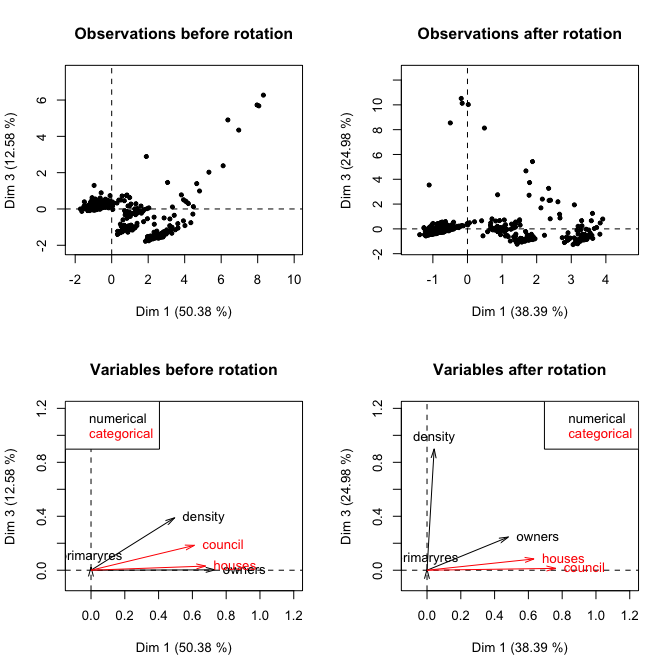It also possible to plot the numerical variables (on the correlation circle) or the levels of the categorical variables.

``````par(mfrow=c(2,2))
plot(res.pcamix,choice="cor", coloring.var=TRUE, leg=TRUE,axes=c(1,3),
posleg="topright", main="Before rotation")
plot(res.pcarot,choice="cor", coloring.var=TRUE, leg=TRUE,axes=c(1,3),
posleg="topright", main="After rotation")
plot(res.pcamix,choice="levels",  leg=TRUE,axes=c(1,3),
posleg="topright", main="Before rotation",xlim=c(-1.5,2.5))
plot(res.pcarot,choice="levels", leg=TRUE,axes=c(1,3),
posleg="topright", main="After rotation",xlim=c(-1.5,2.5))``````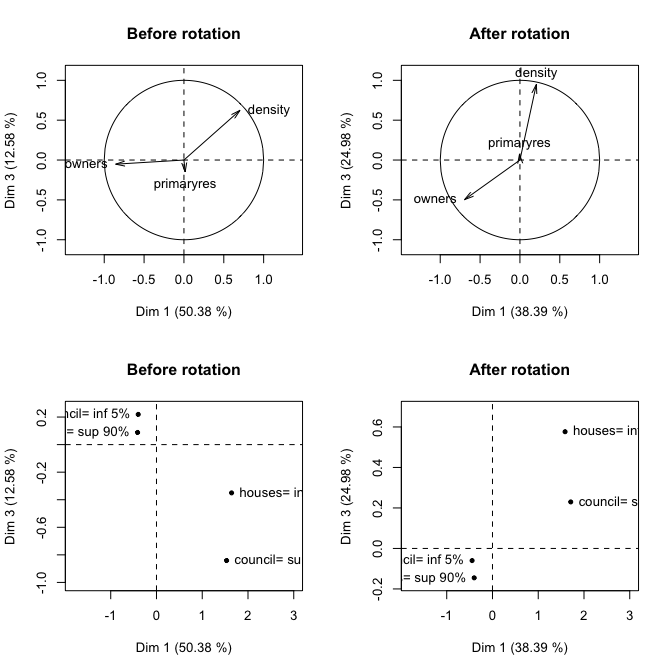### Prediction and plot of new observations.

It is possible, exactly as in PCAmix, to predict the scores of new observations on the principal components after rotation.

``````test <- gironde\$housing[1:10, ]
splitnew <- splitmix(test)
X1new <- splitnew\$X.quanti
X2new <- splitnew\$X.quali
pred.rot <- predict(res.pcarot, X.quanti=X1new, X.quali=X2new)
pred.rot``````
``````##                      dim1.rot   dim2.rot    dim3.rot
## ABZAC               3.2685436  0.3494533 -0.85177749
## AILLAS             -0.7235629  0.1200285 -0.22254455
## AMBARES-ET-LAGRAVE  2.8852451  0.9823515 -0.03451571
## AMBES               1.7220716  1.1590890 -0.78227835
## ANDERNOS-LES-BAINS  0.3423361 -2.6886415  0.90574890
## ARBANATS           -0.6653760  0.4217893  0.13105217
## ARBIS              -0.7668742  0.3099338 -0.23304721
## ARCACHON            1.8825083 -4.4533014  2.36935740
## ARCINS             -0.6896492  0.2060403 -0.09049882``````

An of course these new observations can be plot as supplementary observations on the principal component map of the observations after rotation.

``````plot(res.pcarot,axes=c(1,3),label=FALSE,main="Observations map after rotation")
points(pred.rot[,c(1,3)],col=2,pch=16)
legend("topright",legend = c("train","test"),fill=1:2,col=1:2)``````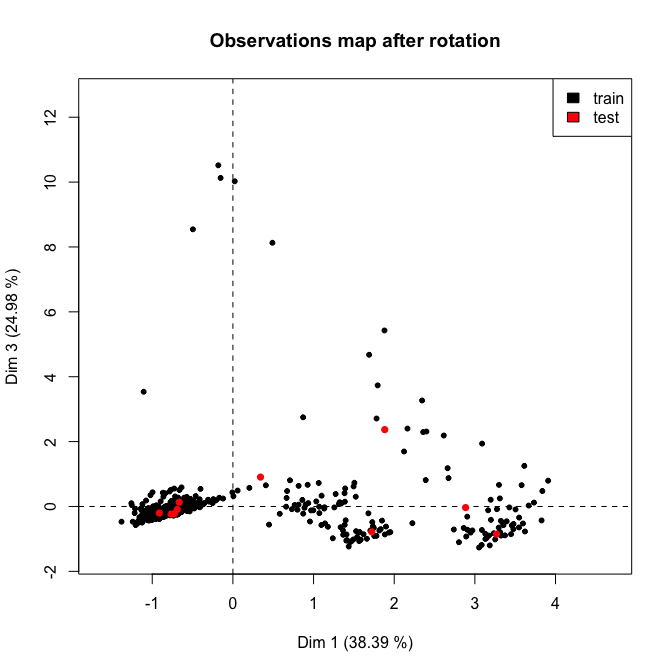# MFAmix

We use here the 4 mixed datatables available in the dataset gironde. This dataset is a list of 4 datatables (one datatable by group).

``````library(PCAmixdata)
data(gironde)
names(gironde)``````
``##  "employment"  "housing"     "services"    "environment"``

Then we apply the function MFAmix to the four datatables concatenated in a single datatable.

``````#Concatenation of the 4 datatables
dat <- cbind(gironde\$employment,gironde\$housing,gironde\$services,gironde\$environment)
index <- c(rep(1,9),rep(2,5),rep(3,9),rep(4,4))
names <- c("employment","housing","services","environment")
res.mfamix<-MFAmix(data=dat,groups=index,
name.groups=names,ndim=3,rename.level=TRUE,graph=FALSE)``````

The object res.mfamix is an object of class MFAmix and contains many numerical results summarized in the S3 print method.

``class(res.mfamix)``
``##  "MFAmix"``
``res.mfamix``
``````## **Results of the Multiple Factor Analysis for mixed data (MFAmix)**
## The analysis was performed on 542 individuals, described by 27 variables
## *Results are available in the following objects :
##
## "name" "description"
## "\$eig" "eigenvalues"
## "\$eig.separate" "eigenvalues of the separate analyses"
## "\$separate.analyses" "separate analyses for each group of variables"
## "\$groups" "results for all the groups"
## "\$partial.axes" "results for the partial axes"
## "\$ind" "results for the individuals"
## "\$ind.partial" "results for the partial individuals"
## "\$quanti" "results for the quantitative variables"
## "\$levels" "results for the levels of the qualitative variables"
## "\$quali" "results for the qualitative variables"
## "\$listvar.group" "list of variables in each group"
## "\$global.pca" "results for the global PCA"``````

### Graphical outputs

The graphical outputs of MFAmix are quite similar to those of PCAmix with some specifity introduced by the knowedge of groups of variables. They are obtained with the method plot of the objects of class MFAmix.

``?plot.MFAmix``
``## Rendering development documentation for 'plot.MFAmix'``
``````par(mfrow=c(2,2))
plot(res.mfamix, choice="cor",coloring.var="groups",leg=TRUE,
main="(a) Numerical variables")
plot(res.mfamix,choice="ind", partial=c("SAINTE-FOY-LA-GRANDE"), label=TRUE,
posleg="topright", main="(b) Observations")
posleg="topright",main="(c) All variables")
plot(res.mfamix, choice="groups", coloring.var="groups",
main="(c) Groups")``````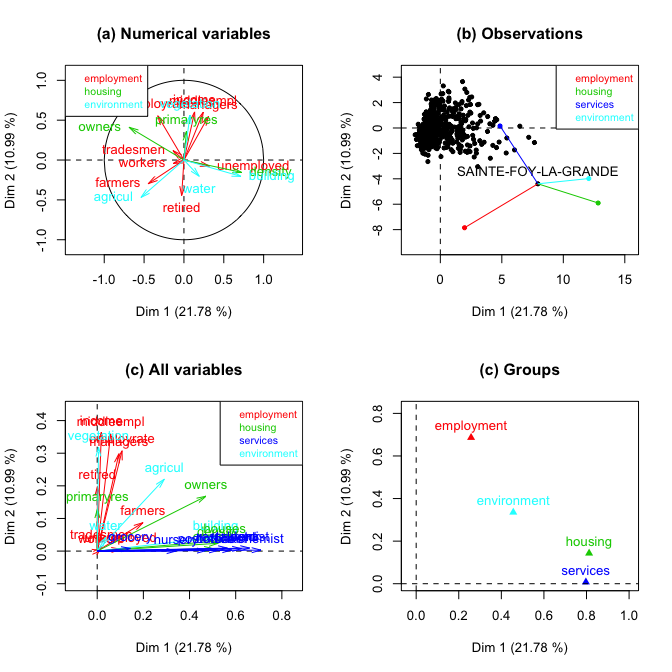Because the correlation circle can be difficult to read with the superposition of the names of some variables, it can be useful to look at the numerical values the most correlated (in absloute value) to the 2 first principal components of PCAmix.

``````coord.var <- res.mfamix\$quanti\$coord[,1:2]
subset(coord.var,abs(coord.var[,1])>0.5,1)``````
``````##               dim 1
## density   0.7237913
## owners   -0.6863817
## building  0.7161893
## agricul  -0.5390967``````
``subset(coord.var,abs(coord.var[,2 ])>0.5,2)``
``````##                dim 2
## managers   0.5463422
## middleempl 0.5992579
## employrate 0.5545209
## income     0.6038040
## vegetation 0.5642491``````

In order to have details on the way the variables of the group services (in blue on the map of all the variables) interpret the first principal component, we look at the map the levels of the categorical variables;

``````plot(res.mfamix, choice="levels", coloring.var="groups",
posleg="bottomleft", main="(c) Levels",xlim=c(-2,4))``````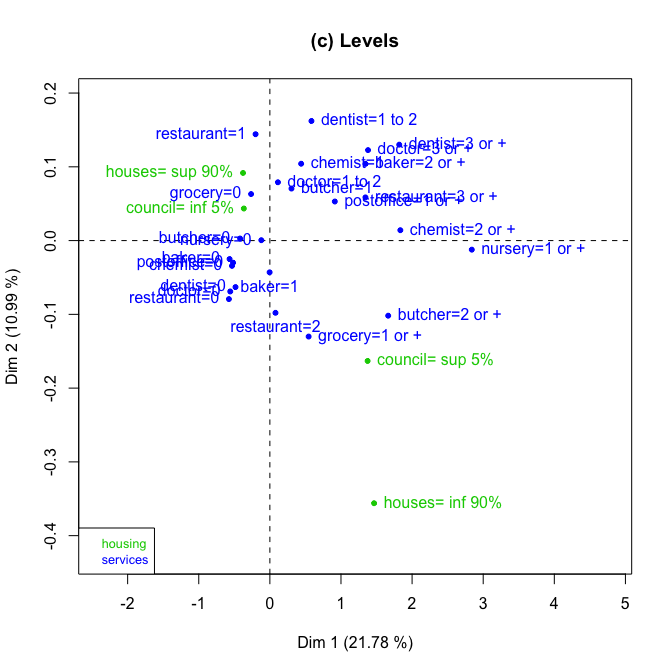### Prediction and plot of new observations

We want to put the municipality SAINTE-FOY-LA-GRANDE as supplementary observation in the MFAmix analysis.

``````sel <- which(rownames(dat)=="SAINTE-FOY-LA-GRANDE")
res.mfamix<- MFAmix(data=dat[-sel,],groups=index,
name.groups=names,rename.level=TRUE,graph=FALSE)
pred <- predict(res.mfamix,dat[sel,,drop=FALSE])
pred[,1:2,drop=FALSE]``````
``````##                          dim1      dim2
## SAINTE-FOY-LA-GRANDE 8.273689 -4.363703``````

It is then possible to plot SAINTE-FOY-LA-GRANDE as an illustrative municipality.

``````plot(res.mfamix,axes=c(1,2),label=FALSE,main="Observations map",
ylim=c(-5,1.5),cex=0.8)
points(pred[,1:2,drop=FALSE],col=2,pch=16)
text(pred,"SAINTE-FOY-LA-GRANDE",col=2,cex=0.7,pos=2)
selplot <- which(res.mfamix\$ind\$coord[,1]>4.2)
text(res.mfamix\$ind\$coord[selplot,1:2],rownames(dat[-sel,])[selplot],col=1,cex=0.7,pos=2)
legend("topright",legend = c("active","illustrative"),fill=1:2,col=1:2)``````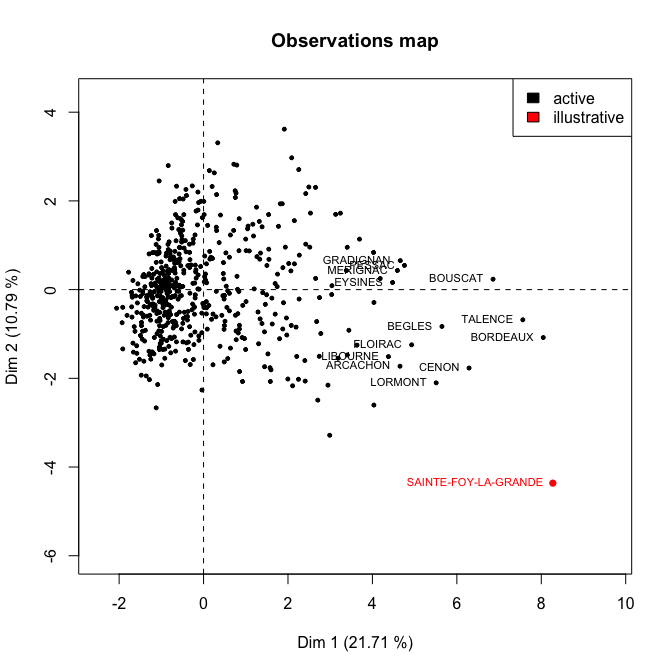### Plot of supplementary groups

Let us for instance apply MFAmix with three groups (employment , services, environment) and add the group housing in supplementary.

``````dat <- cbind(gironde\$employment,gironde\$services,gironde\$environment)
names <- c("employment","services","environment")
mfa <-MFAmix(data=dat,groups=c(rep(1,9),
rep(2,9),rep(3,4)),
name.groups=names,
rename.level=TRUE,graph=FALSE)
mfa.sup <- supvar(mfa,data.sup=gironde\$housing,
groups.sup=rep(1,5),
name.groups.sup="housing.sup",rename.level=TRUE)``````

The group housing can then plotted as supplementary on the maps of MFAmix.

``````#par(mfrow=c(1,2))
plot(mfa.sup,choice="groups",coloring.var="groups")``````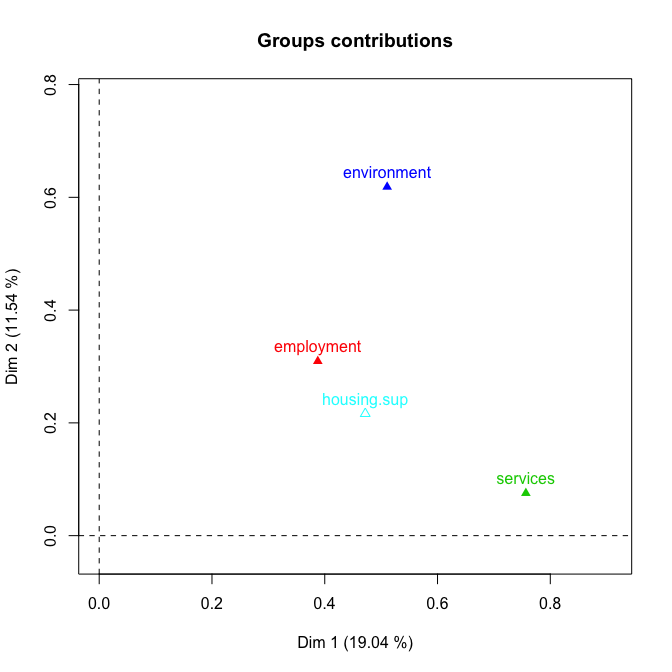``plot(mfa.sup,choice="cor",coloring.var = "groups")``